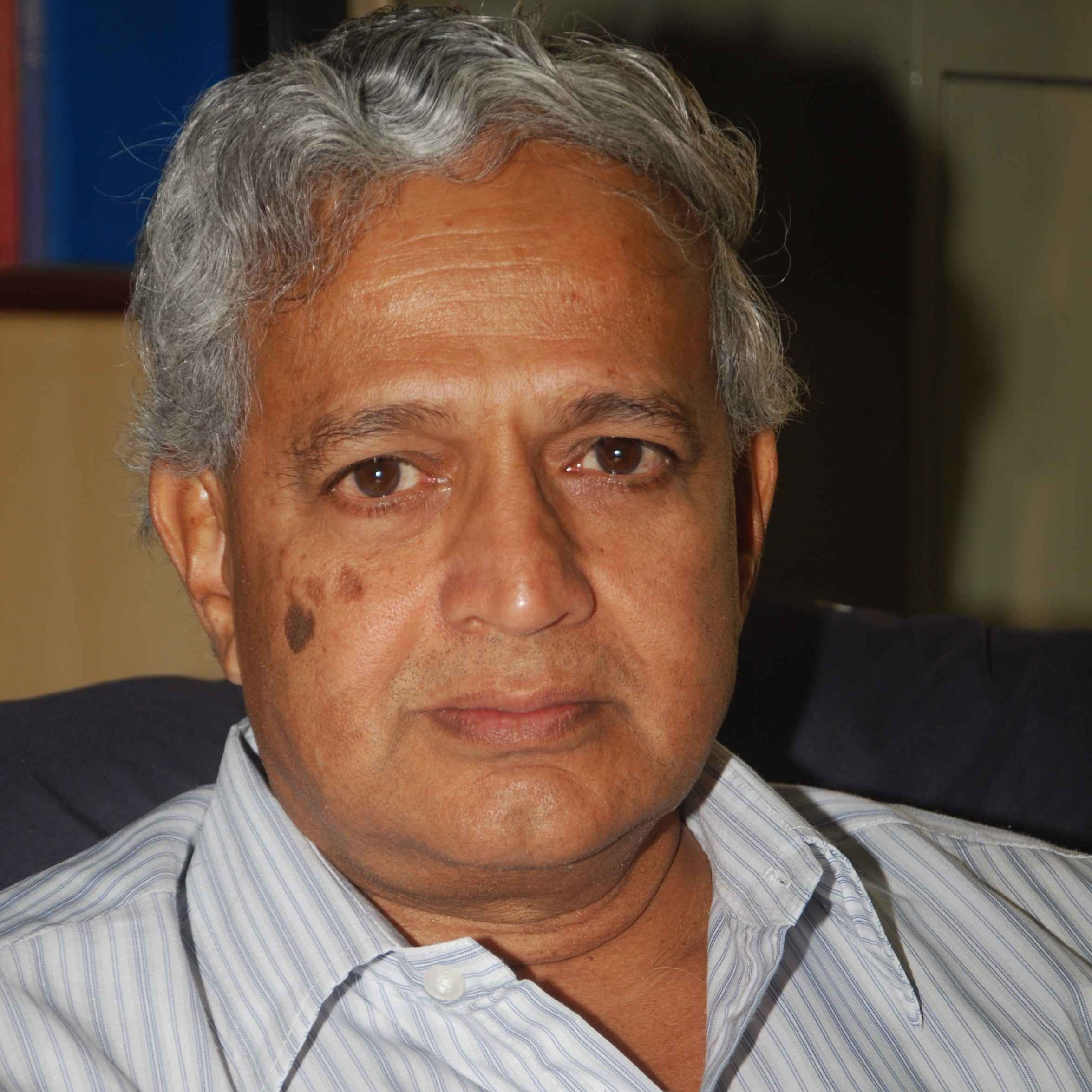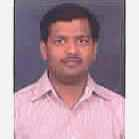X
Electro-thermo-elastic formulation for the analysis of smart structures
Published in
2006
Volume: 15

Issue: 2
Pages: 401 - 416
Abstract
Coupled electro-thermo-elastic equations applicable for the analysis of smart structures with piezoelectric patches/layers have been derived from the fundamental principles of mass, linear momentum, angular momentum, energy and charge conservation. The relevant constitutive equations have been obtained by using the second law of thermodynamics. The interaction of the electric field and polarization introduces distributed non-linear body force in the piezo material, and in addition renders the stress tensor non-symmetric due to distributed couple. Using the linear equations, and applying a layer-by-layer finite element model, the induced electric potential and mechanical deformations in the piezo and non-piezo core material have been obtained for various cases of actuation and sensing of a smart beam under external mechanical, electrical and thermal loadings. The mathematical formulation and the solution technique have been validated by comparing the results of the present study with those available in the literature. It is also shown that piezo patches can be effectively used for shape control. © 2006 IOP Publishing Ltd.
••